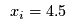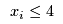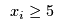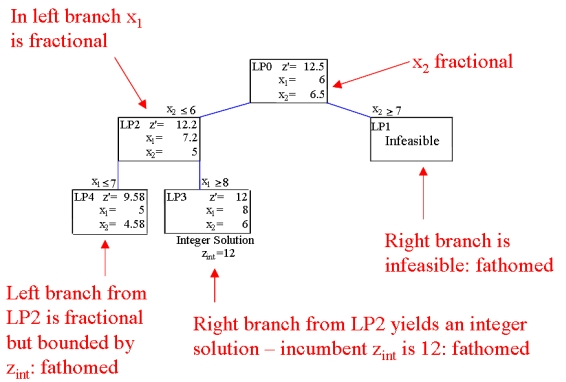# Integer Programming

Integer programmes are almost identical to linear programmes with one very important exception. Some of the decision variables in integer programmes can only have integer values. The variables are known as integer variables. Since most integer programmes contain a mix of real variables (i.e., that can have any real value) and integer variables they are often known as mixed integer programmes. While the change from a linear programming formulation is a minor one, the effect on the solution process is enormous. Integer programmes can be very difficult problems to solve and currently a lot of research is focussing on finding "good" ways to solve integer programmes.

Integer programming, the process of solving a (mixed) integer programme, was originally done using the branch-and-bound process. The branch part of the process eliminated non-integer values for integer variables in the following way:

• Initially, all variables are left as real variables. The problem is solved using linear programming;
• If one of the integer variables in the linear programming solution has a fractional value, e.g.,, then the linear programme is split in two and the fractional region eliminated. This is done by branching on the variable value to get two new linear programmes, e.g., adding the constraintto form one linear programme andto form the other.
• By finding the optimal solution in each of these new linear programmes and comparing we can find the optimal solution for the original problem;
• If either of the new linear programmes has a fractional value for an integer variable then a new branch is needed.

This branching process results in the formation of a branch-and-bound tree (we will discuss the bounding next). Each node in this tree represents a linear programme consisting of the original linear programme and the extra branches added. Eventually all the leaf nodes in the tree will contain solutions where all the integer variables have integer values (an integer solution) and no further branching is needed. All these values can be compared and the best one is the solution to the original integer programme.

Note For mixed integer programmes of any reasonable size this tree could be huge; in fact it grows exponentially as the number of integer variables increases.

The bounding process allows sections of the branch-and-bound tree to be removed from consideration before all the leaf nodes have integer solutions. It relies on the following optimisation principle: Adding constraints to a mathematical programme will result in a deterioration of the optimal objective value.

This means that adding the branching constraints to the linear programmes at the branch-and-bound tree nodes will mean the resulting nodes will have an optimal objective function value that is equal to or worse than the optimal objective function value of the original linear programme. Thus the objective function values get worse the deeper into the tree you look. Since we are finding the integer solution in the branch-and-bound tree with the best objective value, we can use any integer solutions to bound the tree.

The current best integer solution is called the incumbent. After solving a linear programme at a leaf node of the branch-and-bound tree one of the following conditions holds:

• The linear programme is infeasible (no more branching is possible);
• The linear programme solution is an integer solution with a better objective value than the incumbent. The incumbent is replaced with this new solution;
• The linear programme solution has an equal or worse objective than the incumbent. Any nodes created from this node will also have an equal or worse objective than the incumbent. This node is bounded by the incumbent objective;
• The linear programme solution is fractional and has a better objective value than the incumbent. Further branching from this node is necessary to ensure an optimal solution is found.

Only the last condition requires more branching, all the other conditions result in the node becoming fathomed and no more branching is required from that node.

Example of a branch-and-bound tree## Integer Programming with OR Software

To see integer programming in action, check out some of the integer programming case studies:

#### Results from OpsRes web retrieved at 01:47 (GMT)

\usepackage{amsmath} Case Study: Submitted: Operations Research Topics: Application Areas: Contents Problem Description Problem Description Return...
\usepackage{amsmath} Case Study: Submitted: Operations Research Topics: Application Areas: Contents Problem Description Problem Description Return...
\usepackage{amsmath} Case Study: Submitted: Operations Research Topics: Application Areas: Contents Problem Description Problem Description Return...
\usepackage{amsmath} Case Study: Submitted: Operations Research Topics: Application Areas: Contents Problem Description Problem Description Return...
\usepackage{amsmath} Case Study: Submitted: Operations Research Topics: Application Areas: Contents Problem Description Problem Description Return...
\usepackage{amsmath} Case Study: Submitted: Operations Research Topics: Application Areas: Contents Problem Description Problem Description Return...
\usepackage{amsmath} Case Study: Submitted: Operations Research Topics: Application Areas: Contents Problem Description Problem Description Return...
\usepackage{amsmath} Case Study: Submitted: Operations Research Topics: Application Areas: Contents Problem Description Problem Description Return...
\usepackage{amsmath} Case Study: Submitted: Operations Research Topics: Application Areas: Contents Problem Description Problem Description Return...
\usepackage{amsmath} Case Study: Submitted: Operations Research Topics: Application Areas: Contents Problem Description Problem Description Return...
Number of topics: 10

-- TWikiAdminGroup - 20 Feb 2008

Topic revision: r23 - 2018-11-23 - TWikiAdminUserCopyright © 2008-2023 by the contributing authors. All material on this collaboration platform is the property of the contributing authors.
Ideas, requests, problems regarding TWiki? Send feedback